PPBstats is a freely available package based on the R software for Participatory Plant Breeding statisticial analyses. It performs analyses on the data collected during PPB programs at four levels:

• network of seed management,
• agronomic trials,
• organoleptic tests and
• molecular analyses.

The objectives of PPBstats are

1. to have a single package capable of performing several analyses required for PPB programmes with comprehensive documentation, and
2. to create a community working on PPB programmes in order to improve the package, exchange on how to process data from PPB programmes and develop good practices.

## Examples of analysis performed by PPBstats

library(PPBstats)

### Network of seed management

Descriptive analysis can be done regarding:

• unipart network for seed lots analysis
• unipart network for location analysis
• bipart network analysis

Below an example on unipart network for seed lots analysis:

# get data
data(data_network_unipart_sl)

# format data for the package
net_unipart_sl = format_data_PPBstats(
type = "data_network",
data = data_network_unipart_sl,
network_part = "unipart",
vertex_type =  "seed_lots")

# Display outputs
plot(net_unipart_sl, plot_type = "network", organize_sl = TRUE)

## []
## []$network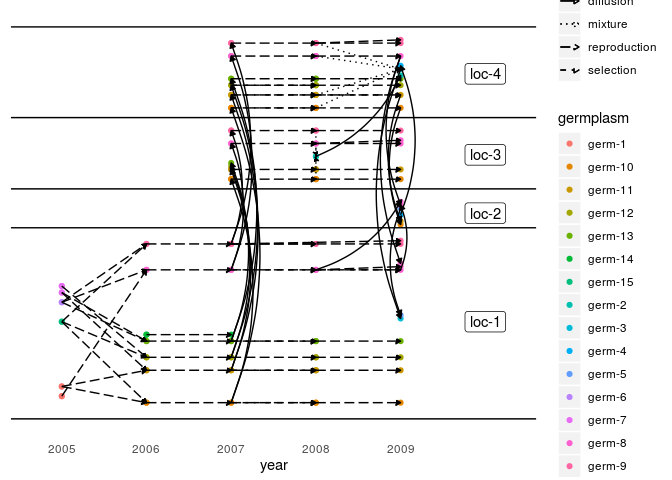Other examples can be found in the book. ### Agronomic trials Statistical analysis can be done • To compare different germplasms on each location for selection: • classic anova, • spatial analysis, • mixed models, • bayesian hierarchical model intra-location. • To study response of germplasm over several locations for selection: • AMMI and GGE, • bayesian hierarchical model G×E. • To study specific research questions on one farm or more • response to selection • local adaptation with two models: home away and local foreign • intra germplasm variance • To run multivariate analysis Below an example on GGE model: # get data data(data_model_GxE) # format data for the package data_model_GxE = format_data_PPBstats(data_model_GxE, type = "data_agro") Once the data are formated, the model is run # run the GGE model out_gge = model_GxE(data_model_GxE, variable = "y1", gxe_analysis = "GGE") Then, check it, for example with residuals # check if the model went well out_check_gge = check_model(out_gge) p_out_check_gge = plot(out_check_gge) p_out_check_gge$residuals

## $histogram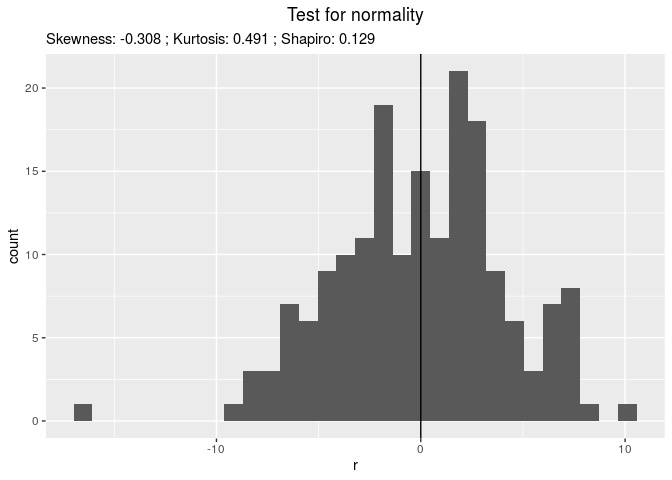## ##$qqplot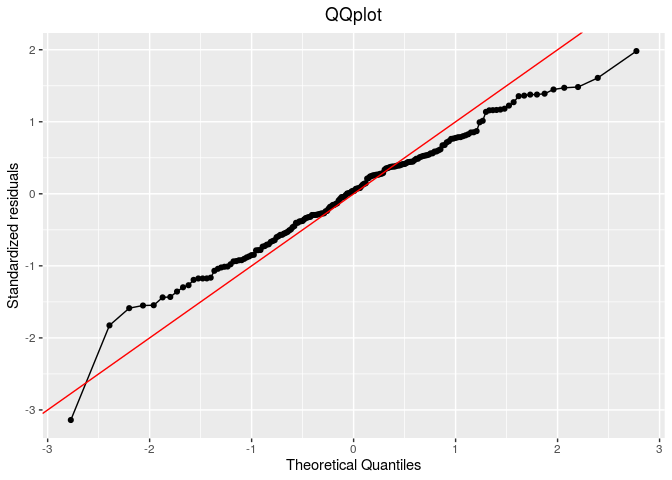##
## $points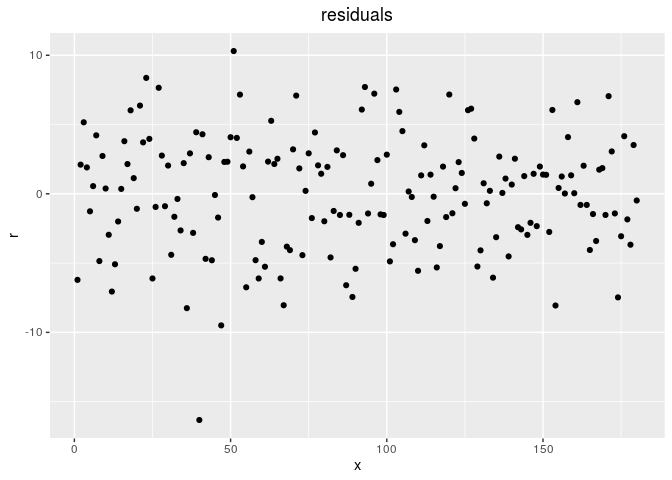Then, compare means # get mean comparisons out_mean_comparisons_gge = mean_comparisons(out_check_gge, p.adj = "bonferroni") p_out_mean_comparisons_gge = plot(out_mean_comparisons_gge) p_out_mean_comparisons_gge$germplasm$1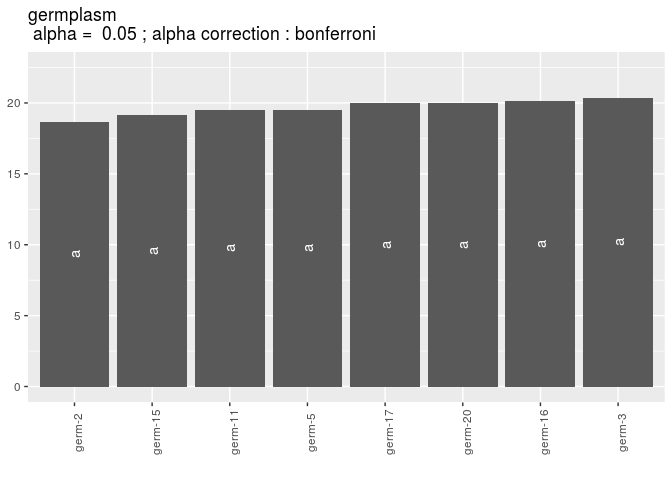Then, look at biplot # get biplot out_biplot_gge = biplot_data(out_check_gge) p_out_biplot_gge = plot(out_biplot_gge) p_out_biplot_gge$biplot$which_won_where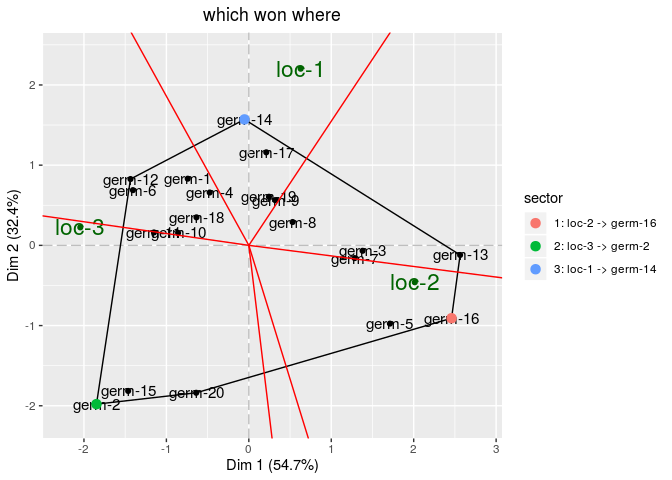### Organoleptic tests Sensory analysis can be done on • hedonic analysis • napping analysis • rank analysis Below an example on hedonic analysis # get data data(data_hedonic) # format data for the package data_hedonic = format_data_PPBstats(data_hedonic, type = "data_organo_hedonic", threshold = 2) # descriptive analysis p_note = plot(data_hedonic, plot_type = "boxplot", x_axis = "germplasm", in_col = "location", vec_variables = "note" ) p_note$note$germplasm-1|location-1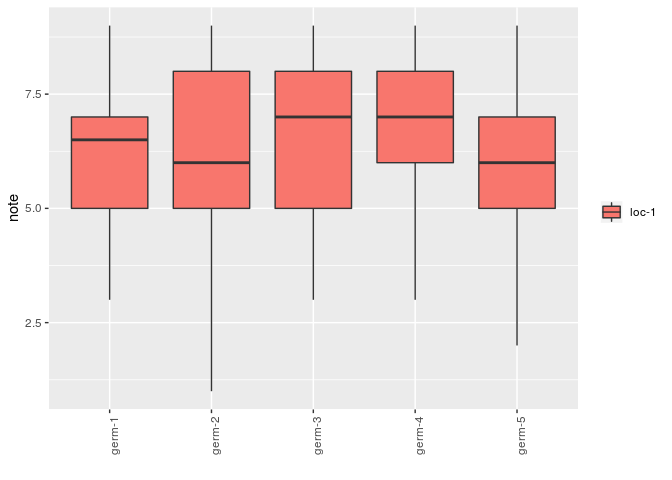descriptors = data_hedonic$descriptors

p_des = plot(data_hedonic, plot_type = "radar", in_col = "germplasm",
vec_variables = descriptors
)
p_des\$all-variables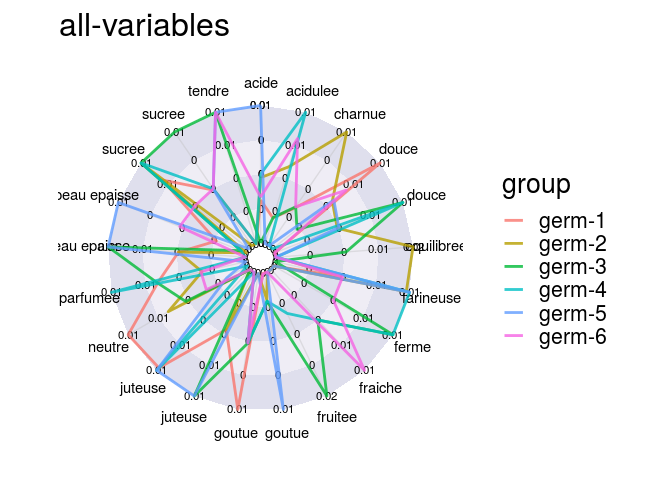A model can also be run.

### Molecular analyses

Under development …

## Tutorial

A full tutorial of PPBstats is available in the book on the website.

## Mailing list ppbstats@framalistes.org

Exchange information about the R package PPBstats

You can subscribe to this mailing list to have news on the R package PPBstats and share questions and remarks